# Closed set

In mathematics, a set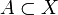$A \subset X$, where (X,O) is some topological space, is said to be closed if its complement in X, the set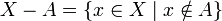$X-A=\{x \in X \mid x \notin A\}$, is open. The empty set and the set X itself are always closed sets. The finite union and arbitrary intersection of closed sets are again closed.

## Examples

1. Let X be the open interval (0, 1) with the usual topology induced by the Euclidean distance. Open sets are then of the form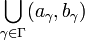$\bigcup_{\gamma \in \Gamma} (a_{\gamma},b_{\gamma})$
where 0 < aγ < bγ < 1, and Γ is an arbitrary index set. The definition now implies that closed sets are of the form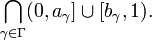$\bigcap_{\gamma \in \Gamma} (0,a_{\gamma}]\cup [b_{\gamma},1).$.
2. As a more interesting example, consider the function space C[a,b] (with a < b). This space consists of all real-valued continuous functions on the closed interval [a, b] and is endowed with the topology induced by the norm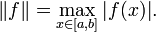$\|f\| = \max_{x \in [a,b]} |f(x)|.$
In this topology, the sets$A = \big\{ f \in C[a,b] \mid \min_{x \in [a,b]} f(x) > 0 \}$
and$B = \big\{ f \in C[a,b] \mid \min_{x \in [a,b]} f(x) < 0 \}$
are open sets while the sets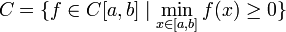$C = \big\{ f \in C[a,b] \mid \min_{x \in [a,b]} f(x) \ge 0 \}$
and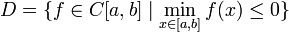$D = \big\{ f \in C[a,b] \mid \min_{x \in [a,b]} f(x) \le 0 \}$
are closed (the sets C and D are the closure of the sets A and B respectively).Some content on this page may previously have appeared on Citizendium.